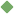factor_analysis 1. [ noun ] (mathematics,statistics) any of several methods for reducing correlational data to a smaller number of dimensions or factors; beginning with a correlation matrix a small number of components or factors are extracted that are regarded as the basic variable that account for the in Related terms: correlational_analysis statistics To share this definition press "text" (Facebook, Twitter) or "link" (blog, mail) then paste text link Similar spelling: factor_analyse   factor_analyze   factor_analytic   factor_analytical   factor_of_safety